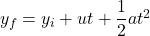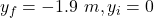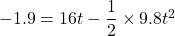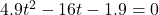Question

A student standing on the ground throws a ball straight up. The ball leaves the student’s hand with a speed of 16.0 m/s when the hand is 1.90 m above the ground. You may want to review (Pages 49 – 51) . For help with math skills, you may want to review: Quadratic Equations For general problem-solving tips and strategies for this topic, you may want to view a Video Tutor Solution of Time in the air for a tossed ball. Part A How long is the ball in the air before it hits the ground

1.t = 3.38 s

Explanation:

We have,

Initial speed of the ball that leaves the student’s hand is 16 m/s

Initially, the hand is 1.90 m above the ground.

It is required to find the time for which the ball in the air before it hits the ground. We can use the equation of kinematics as :Here,and a=-g

The equation become:After rearranging we get the above equation as :It is a quadratic equation, we need to find the value of t. On solving the above equation, we get :

t = -0.115 s and t = 3.38 s (ignore t = -0.115 s )

So, the ball is in air for 3.38 seconds before it hits the ground.